# How to Remove the Legend in Matplotlib?

Matplotlib is one of the most popular data visualization libraries present in Python. Using this matplotlib library, if we want to visualize more than a single variable, we might want to explain what each variable represents. For this purpose, there is a function called legend() present in matplotlib library. This legend is a small area on the graph describing what each variable represents.

In order to remove the legend, there are four ways. They are :

• Using .remove()
• Using .set_visible()
• Fix legend_ attribute of the required Axes object = None
• Using label=_nolegend_

Method 1: Using .remove()

Example 1: By using ax.get_legend().remove() method, legend can be removed from figure in matplotlib.

## Python3

 `import` `numpy as np` `import` `matplotlib.pyplot as plt`   `x ``=` `np.linspace(``-``3``, ``3``, ``100``)` `y1 ``=` `np.power(x, ``2``)` `y2 ``=` `np.power(x, ``3``)`   `fig, ax ``=` `plt.subplots()`   `ax.plot(x, y1, c ``=` `'r'``,label ``=` `'x^2'``)` `ax.plot(x, y2, c ``=` `'g'``,label ``=` `'x^3'``)`   `leg ``=` `plt.legend()`   `ax.get_legend().remove()`   `plt.show()`

Output :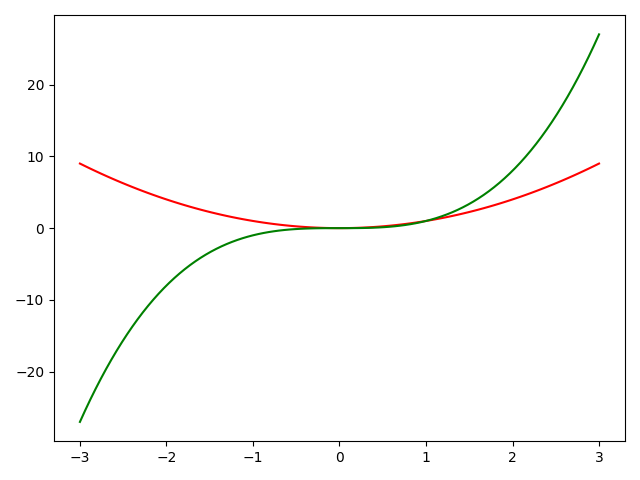We can see that there is no legend in the above figure.

Example 2: More than one subplots :

In the case of more than one subplot, we can mention the required subplot object for which we want to remove the legend. Here, we have written axs.get_legend().remove() which means we are removing legend for second subplot specifically.

## Python3

 `import` `numpy as np` `import` `matplotlib.pyplot as plt`   `x ``=` `np.linspace(``-``3``, ``3``, ``100``)` `y1 ``=` `np.power(x, ``2``)` `y2 ``=` `np.power(x, ``3``)`   `fig, axs ``=` `plt.subplots(``2``, ``1``)`   `axs[``0``].plot(x, y1, c ``=` `'r'``,label ``=` `'x^2'``)` `axs[``1``].plot(x, y2, c ``=` `'g'``,label ``=` `'x^3'``)`   `axs[``0``].legend(loc ``=` `'upper left'``)` `axs[``1``].legend(loc ``=` `'upper left'``)`   `axs[``1``].get_legend().remove()`     `plt.show()`

Output :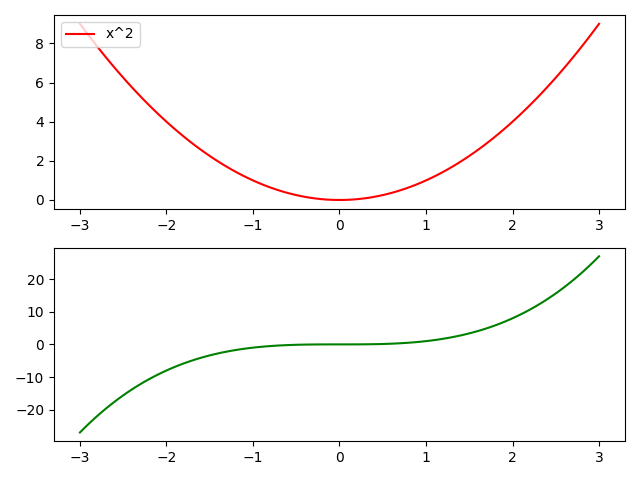In the above figure, we removed the legend for the second subplot specifically. The first subplot will still have a legend.

Method 2: Using set_visible()

Example 1: By using ax.get_legend().set_visible(False) method, legend can be removed from figure in matplotlib.

## Python3

 `import` `numpy as np` `import` `matplotlib.pyplot as plt`   `x ``=` `np.linspace(``-``3``, ``3``, ``1000``)` `y1 ``=` `np.sin(x)` `y2 ``=` `np.cos(x)`   `fig, ax ``=` `plt.subplots()`   `ax.plot(x, y1,c ``=` `'r'``,label ``=` `'Sine'``)` `ax.plot(x, y2,c ``=` `'g'``,label ``=` `'Cosine'``)`   `leg ``=` `plt.legend()`   `ax.get_legend().set_visible(``False``)`   `plt.show()`

Output :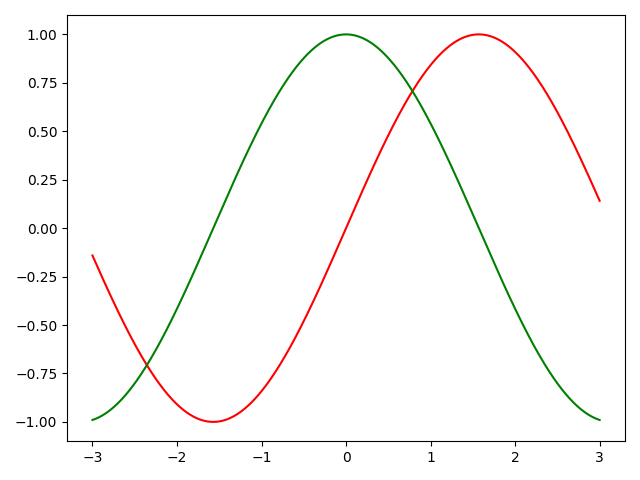We can see that there is no legend in the above figure.

Example-2. More than one subplots  :

In case of more than one subplot, we can mention the required subplot object for which we want to remove the legend. Here, we have written axs.get_legend().set_visible(False) which means we are removing legend for second subplot specifically.

## Python3

 `import` `numpy as np` `import` `matplotlib.pyplot as plt`   `x ``=` `np.linspace(``-``3``,``3``,``1000``)` `y1 ``=` `np.sin(x)` `y2 ``=` `np.cos(x)`   `fig, axs ``=` `plt.subplots(``2``,``1``)`   `axs[``0``].plot(x,y1,c``=``'r'``,label ``=` `'Sine'``)` `axs[``1``].plot(x,y2,c``=``'g'``,label ``=` `'Cosine'``)`   `axs[``0``].legend(loc``=``'upper left'``)` `axs[``1``].legend(loc``=``'upper left'``)`   `axs[``1``].get_legend().set_visible(``False``)`     `plt.show()`

Output :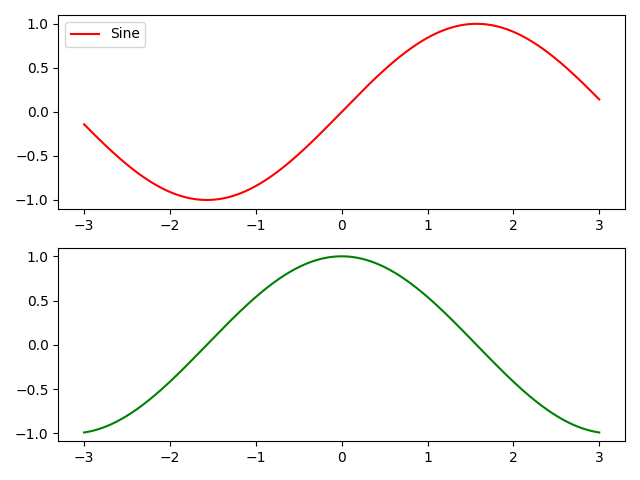In the above figure, we removed legend for the second subplot specifically. The first subplot will still have legend.

Method 3: Fix legend_ attribute of the required Axes object = None :

Example 1: By using ax.legend_ = None, legend can be removed from figure in matplotlib.

## Python3

 `import` `numpy as np` `import` `matplotlib.pyplot as plt`   `x ``=` `np.linspace(``-``3``, ``3``, ``1000``)` `y1 ``=` `np.sin(x)` `y2 ``=` `np.cos(x)`   `fig, ax ``=` `plt.subplots()`   `ax.plot(x, y1,c ``=` `'r'``,label ``=` `'Sine'``)` `ax.plot(x, y2,c ``=` `'g'``,label ``=` `'Cosine'``)` `leg ``=` `plt.legend()` `ax.legend_ ``=` `None`   `plt.show()`

Output:We can see that there is no legend in the above figure.

Example 2: More than one subplot:

In the case of more than one subplot, we can mention the required subplot object for which we want to remove the legend. Here, we have written axs.legend_ = None which means we are removing legend for the first subplot specifically.the

## Python3

 `import` `numpy as np` `import` `matplotlib.pyplot as plt`   `x ``=` `np.linspace(``-``3``, ``3``, ``1000``)` `y1 ``=` `np.sin(x)` `y2 ``=` `np.cos(x)`   `fig, axs ``=` `plt.subplots(``2``, ``1``)`   `axs[``0``].plot(x, y1, c ``=` `'r'``,label ``=` `'Sine'``)` `axs[``1``].plot(x, y2,c ``=` `'g'``,label ``=` `'Cosine'``)` `axs[``0``].legend(loc ``=` `'upper left'``)` `axs[``1``].legend(loc ``=` `'upper left'``)` `axs[``0``].legend_ ``=` `None`   `plt.show()`

Output: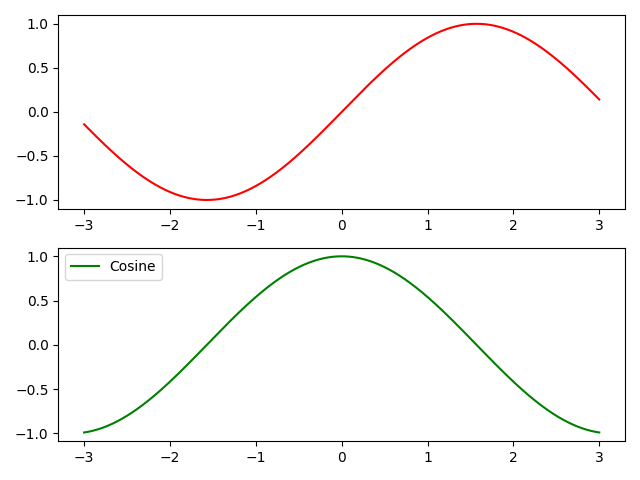In the above figure, we removed legend for the first subplot specifically. The second subplot will still have legend.

Method 4: Using label = _legend_

Example 1: By sending label = ‘_nolegend_’ argument in ax.plot(), legend can be removed from figure in matplotlib.

## Python3

 `import` `numpy as np` `import` `matplotlib.pyplot as plt`   `x ``=` `np.linspace(``-``3``, ``3``, ``100``)` `y1 ``=` `np.power(x, ``2``)` `y2 ``=` `np.power(x, ``3``)`   `fig, ax ``=` `plt.subplots()`   `ax.plot(x, y1, c ``=` `'r'``,label ``=` `'_nolegend_'``)` `ax.plot(x, y2,c ``=` `'g'``,label ``=` `'_nolegend_'``)`   `leg ``=` `plt.legend()`   `plt.show()`

Output: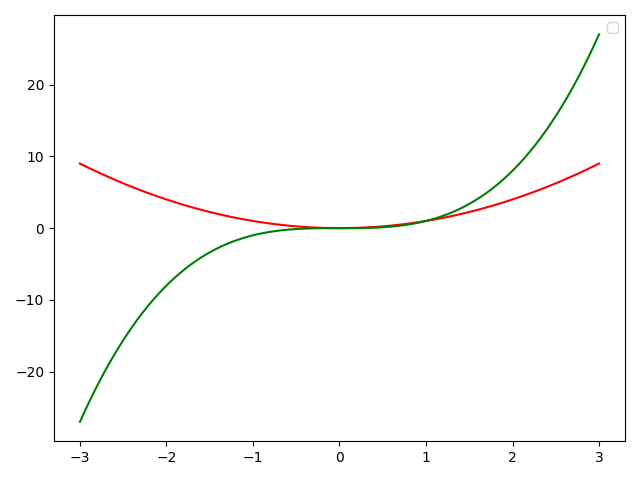Example-2. More than one subplots  :

In case of more than one subplot, we can mention the required subplot object for which we want to remove the legend. Here, we have written axs.plot(x,y1,c=’r’,label = ‘_nolegend_’) which means we are removing legend for first subplot specifically.

## Python3

 `import` `numpy as np` `import` `matplotlib.pyplot as plt`   `x ``=` `np.linspace(``-``3``,``3``,``100``)` `y1 ``=` `np.power(x,``2``)` `y2 ``=` `np.power(x,``3``)`   `fig, axs ``=` `plt.subplots(``2``,``1``)`   `axs[``0``].plot(x,y1,c``=``'r'``,label ``=` `'_nolegend_'``)` `axs[``1``].plot(x,y2,c``=``'g'``,label ``=` `'x^3'``)`   `axs[``0``].legend(loc``=``'upper left'``)` `axs[``1``].legend(loc``=``'upper left'``)`     `plt.show()`

Output :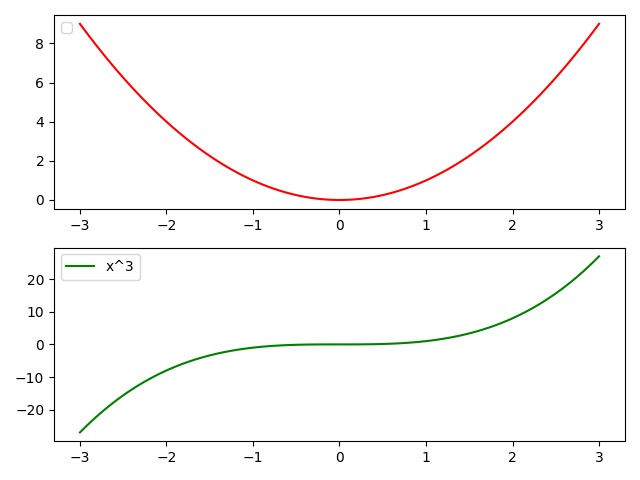In the above figure, we removed legend for the first subplot specifically. The second subplot will still have legend.

Whether you're preparing for your first job interview or aiming to upskill in this ever-evolving tech landscape, GeeksforGeeks Courses are your key to success. We provide top-quality content at affordable prices, all geared towards accelerating your growth in a time-bound manner. Join the millions we've already empowered, and we're here to do the same for you. Don't miss out - check it out now!

Previous
Next